# ML Aggarwal Class 8 Solutions for ICSE Maths Chapter 1 Rational Numbers Ex 1.5

## ML Aggarwal Class 8 Solutions for ICSE Maths Chapter 1 Rational Numbers Ex 1.5

Question 1.
Represent the following rational numbers on the number line:
(i) $$\frac { 11 }{ 4 }$$
(ii) 4$$\frac { 3 }{ 5 }$$
(iii) $$\frac { -9 }{ 7 }$$
(iv) $$\frac { -2 }{ -5 }$$
Solution: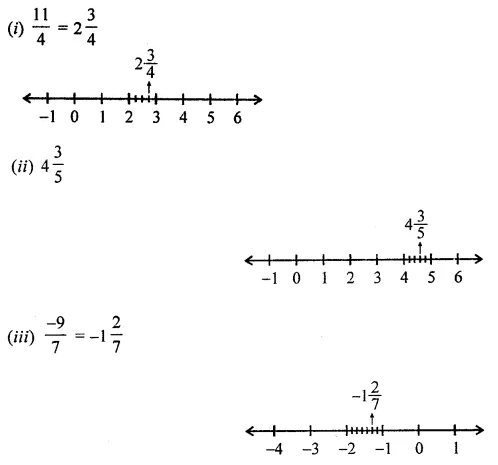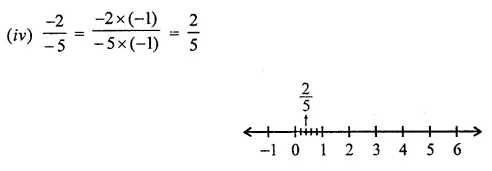Question 2.
Write the rational numbers for each point labelled with a letter:Solution:Question 3.
Find twenty rational numbers between $$\frac { -3 }{ 7 }$$ and $$\frac { 2 }{ 3 }$$
Solution:Question 4.
Find six rational numbers between $$\frac { -1 }{ 2 }$$ and $$\frac { 5 }{ 4 }$$
Solution: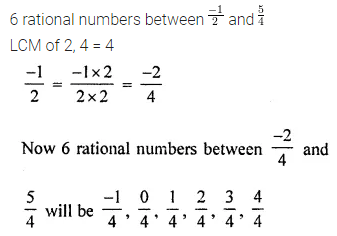Question 5.
Find three rational numbers between -2 and -1.
Solution:Question 6.
Write ten rational numbers which are greater than 0.
Solution: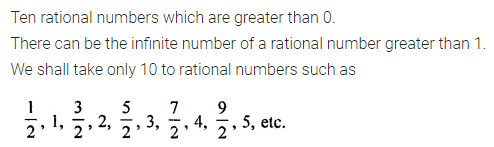Question 7.
Write five rational numbers which are smaller than -4.
Solution: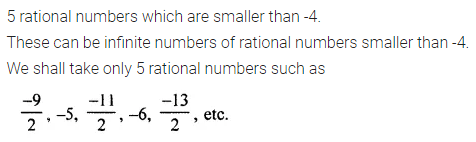Question 8.
Identify the rational number which is different from the other three. Explain your reasoning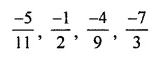Solution: